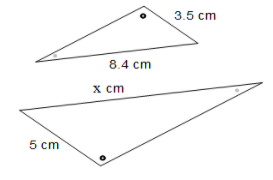# Finding Missing Side Lengths

These triangles are scaled copies of each other. If x = 4 and y = 5 find a and b.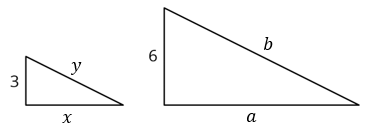These triangles are scaled copies of each other. Select all true equations.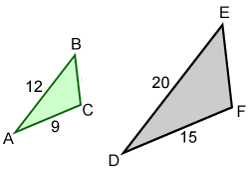Select all that apply
• A
• B
• C
• D
• E
• F

Solve the equation.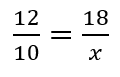Solve the equation.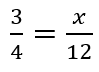Solve the equation.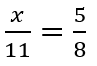These triangles are scaled copies of each other. Select all the equations that could be used to solve for x.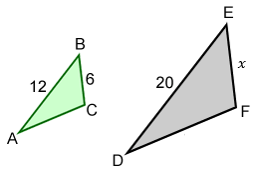Select all that apply
• A
• B
• C
• D
• E

These triangles are scaled copies of each other. Find the value of x.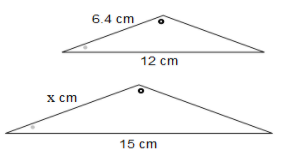These triangles are scaled copies of each other. Find the value of x.